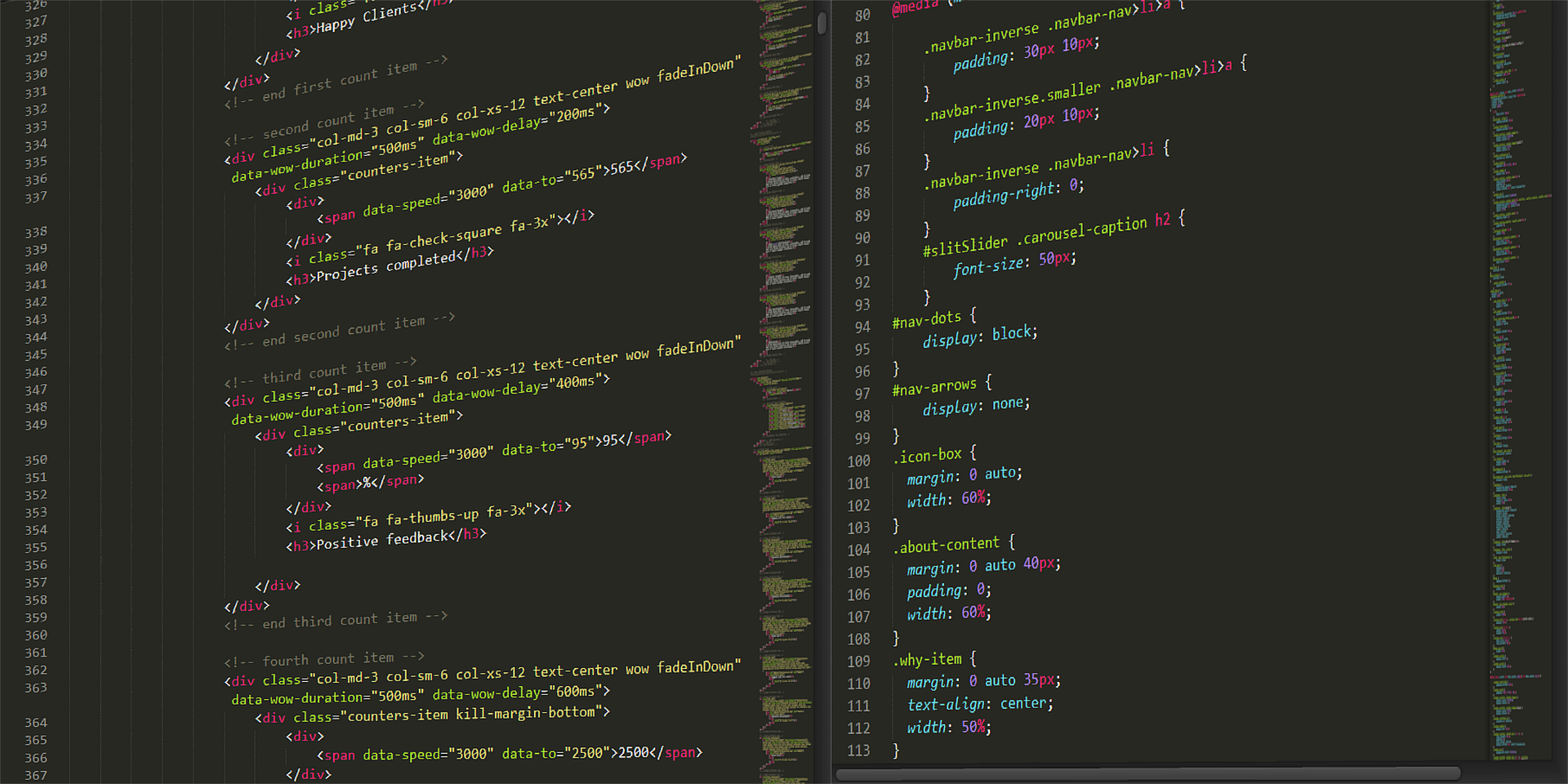Posted on

No two sequences of bits that each have even parity can differ in only one position. For if two such bit sequences differ in exactly one position, then one has exactly one more 1 than the other. Thus, one sequence must have odd parity and the other even parity, contradicting our assumption that both have even parity. We conclude that addition of a parity bit to make the number of 1’s even serves to create an error-detecting code for characters. The parity-bit scheme is quite “efficient,” in the sense that it allows us to transmit many different characters. Note that there are 2n different sequences of n bits, since we may choose either of two values (0 or 1) for the first position, either of two values for the second position, and so on, a total of 2× 2 × · · ·× 2 (n factors) possible strings. Thus, we might expect to be able to represent up to 28 = 256 characters with eight bits. However, with the parity scheme, we can choose only seven of the bits; the eighth is then forced upon us.We can thus represent up to 27 , or 128 characters, and still detect single errors. That is not so bad; we can use 128/256, or half, of the possible 8-bit codes as legal codes for characters, and still detect an error in one bit. Similarly, if we use sequences of n bits, choosing one of them to be the parity bit, we can represent 2n−1 characters by taking sequences of n−1 bits and prefixing the suitable parity bit, whose value is determined by the other n − 1 bits.

Since there are 2n sequences of n bits, we can represent 2n−1/2 n, or half the possible number of characters, and still detect an error in any one of the bits of a sequence. Is it possible to detect errors and use more than half the possible sequences of bits as legal codes? Our next example tells us we cannot. The inductive proof uses a statement that is not true for 0, and for which we must choose a larger basis, namely 1.

𐌢